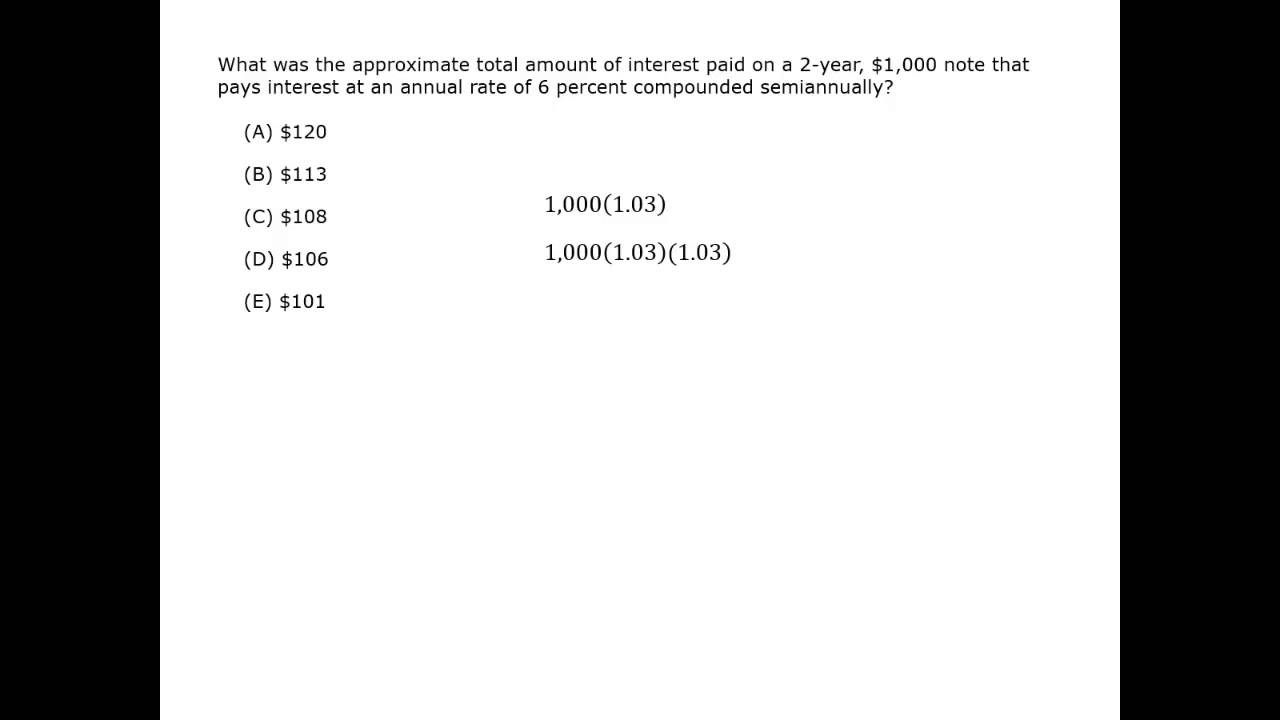#### Simple Interest

The formula for calculating simple interest is:

I = P r t

The amount of interest I is the dollars earned or paid to the investor.

The principal P is the amount of money invested.

The interest rate r is the annual interest rate expressed as a decimal value.

The time period t is measured in years.

A student invests $1,000 at 10% simple interest for the summer (3 months). How much interest does the student earn? ### Solution Use the simple interest formula. I = Prt The principal is$1,000. The interest rate of 10% per year is 0.10. The time is 3 months, so 3/12 of a year.

I = 1,000(0.10)( 3/12 )

I = 100(1/4) = 25

### Solution

First, note that $1000 is added EACH year, so$5000 is invested.

Simple interest means that no interest is paid on accrued interest, only on the principal (which in this case grows by $1000 every year). The first$1000 will earn interest for 5 years.
I = 1000(0.08)(5) = 400
The first $1000 will earn$400.
So after 5 years, the amount in the account from this first $1000 is 1000 + 400 =$1400.

The second $1000 will earn interest for 4 years for a total of$320.
The amount in the account from this $1000 is 1000 + 320 =$1320.

The third $1000 will earn interest for 3 years for a total of$240.
The amount in the account from this $1000 is 1000 + 240 =$1240.

The fourth $1000 will earn$160 and be worth $1160. The last$1000 will earn $80 and be worth$1080.

The money in the account after 5 years is the total of these five values:

$1400 +$1320 + $1240 +$1160 + $1080 =$6200

#### Compound Interest

The formula for calculating compound interest is:

A = P (1 + r/n)nt

As before, the principal P is the amount of money invested. The interest rate r is the annual interest rate expressed as a decimal value. The time period t is measured in years.

This formula gives the value of the account A, not the interest earned. The new component is n, which is how often the interest is paid or compounded per year.

An account has $1500 invested with semi-annual interest of 4%. How much will the account earn in its first year? ### Solution The principal is$1500. The interest rate is 4%. Interest is paid twice a year, so n = 2. The time is 1 year.

A = P (1 + r/n)nt

A = 1500 (1 + 0.04/2)2 × 1

A = 1500(1.02)2 = 1500(1.0404) = 1560.60

The account now has $1560.60. But be careful, the question asks how much was earned, which is: 1560.60 – 1500 = 60.60 The account earned$60.60.

On the GMAT, you may encounter a question where it seems like compound interest would take too long to calculate. By looking at the answer choices you can determine if an estimate will work. Part (c) in the Example below shows how you can estimate and use critical reasoning to find the correct answer.

An account has $4000 invested and earns 5%. How much will the account be worth after one year if: (a) the interest is simple interest (b) the interest is compounded quarterly (c) the interest is compounded daily ### Solution (a) The formula for simple interest is I = Prt I = 4000(0.05)(1) = 200 The account earned$200 in interest, so the new value is $4200. (b) The formula for compound interest is A = P (1 + r/n)nt The interest is compounded quarterly for a year, so n = 4 and t = 1. A = 4000 (1 + 0.05/4)4 = 4000(1.0125)4 ≈ 4000(1.05) = 4204 The new value is$4204, so the account earned $204 in interest. (c) The formula for compound interest is A = P (1 + r/n)nt The interest is compounded daily for a year, so n = 365 and t = 1. A = 4000 (1 + 0.05/365)365 = 4000(1.00014)365 ≈ 4000(1.0524) = 4210 The new value is$4210, so the account earned $210 in interest. If this was a multiple-choice question, you could look at the answer choices to see how accurate the answer needed to be. Let’s say the answer options are: (A)$200   (B) $210 (C)$4182   (D) $4200 (E)$4210

You could easily calculate that the value with simple interest would be \$4200. Since you know that the amount with compound interest must be greater, the only possible answer choice is (E) and there is no need to do that calculation.

#### Interest

Best viewed in landscape mode

2 questions with video explanations

100 seconds per questionBefore attempting these problems, be sure to review this section on data sufficiency questions.